# Test: Mathematical Logic- 1

## 10 Questions MCQ Test GATE Electrical Engineering (EE) 2023 Mock Test Series | Test: Mathematical Logic- 1

Description
Attempt Test: Mathematical Logic- 1 | 10 questions in 30 minutes | Mock test for GATE preparation | Free important questions MCQ to study GATE Electrical Engineering (EE) 2023 Mock Test Series for GATE Exam | Download free PDF with solutions
QUESTION: 1

### Which of the following is/are tautology:​

Solution:

(a) (a v b)→(b ∧ c)
= (a + b)'+ bc
= a' b' + bc
Therefore, ((a v b) → (b ∧ c)) is contingency and not tautology.
(b) (a ∧ b) →(b v c)
= ab → b + c
= (ab)' + b + c
= a' + b' + b + c
= a' + 1 + c
= 1
So ((a ∧ b) →(b v c)) is tautology,
(c) (a v b)→ (b → c)
= (a + b) → (b' + c)
= (a+ b)' + b' + c
= a' b' + b' + c
= b' + c
So ((a v b) → (b → c)) is contingency but not tautology.
(d) (a → b) → (b→ c)
= (a' + b) → (b' + c)
= (a' + b) + b' + c
= ab' + b' + c
= b' + c
Therefore; ((a → b) → (b → c)} is contingency but not tautology.

QUESTION: 2

### ​If the proposition¬ p ⇒ q is true, then the truth value of the proposition ¬ p v (p ⇒ q), where ¬ is negation, 'v' is inclusive or and '⇒’ is implication, is

Solution: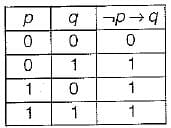Now since ¬ p → q is given true, we reduce the truth table as follows:In the reduced truth table we need to find the truth value of ¬ pv(p→q} = p' +(p → q)
≡ p' + p' + q ≡ p' + q
The truth value of p' + q in the reduced truth table is given below: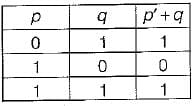Since in the reduced truth table also, the given expression is sometimes true and sometimes false, therefore the truth value of proposition ¬p v (p → q) can not be determined.

QUESTION: 3

### Let a, b, c, d be propositions. Assume that the equivalence a ⇔ ( bv ¬ b) and b ⇔ c hold. Then the truth-value of the formula (a ∧ b) → (a ∧ c) v d is always

Solution:

a ⇔ (b v ¬ b )
a ⇔ 4 True
So a is true, i.e. a = 1
b ⇔ c holds. So b = c
Now the given expression is
(a ∧ b) → ((a ∧ c) v d) ≡ (a - b ) → ((a . c) + d)
Putting a = 1 in above expression we get
1 • b → ((1 • c) + d)
≡ b→ c + d
≡ b' + c+ d
Now putting b = c in above expression we get
≡ c' + c+ d=1 + d=1
So the expression is always true.

QUESTION: 4

The following propositional statement is
(P → (Q v R)) → (( P ∧ Q) → R)

Solution:

(P → (Q v R)) → ((P ∧ Q) → R)
≡ (P→ Q+ R) → (PQ → R)
≡ [P' + Q + R] → [(PQ)' + R]
≡ [P' + Q + R] → [P' + Q' + R]
≡ (P' + Q + R)' + P' + Q' + R
≡ PQ' R' + P' + Q' + R
≡ Q' + Q' PR' + P' + R
≡ Q' + P' + R (by absorption law)
Which is a contingency (i.e. satisfiable but not valid).

QUESTION: 5

A logical binary relation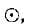is defined as follows: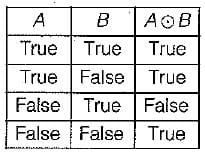Let ~ be the unary negation (NOT) operator, with higher precedence, thanWhich one of the following is equivalent to A ∧ B?

Solution:

By using min terms we can define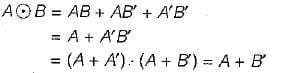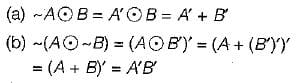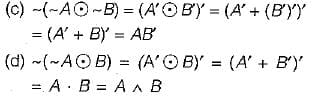∴ Only, choice (d) = A ∧ B
[Note: This problem can also be done by constructing truth table for each choice and - comparing with truth table for A ∧ B]

QUESTION: 6

Which of the following is TRUE about formulae in Conjunctive Normal Form?

Solution:

In conjunction normal form, for any particular assignment of truth values, all except one clause will always evaluate to true. So, the proportion of clauses which evaluate to true to the total number of clauses is equal to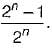Now putting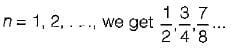All of these proportions are ≥ 1/2 and so choice (a) atleast half of the clauses evaluate to true, is. the correct answer.

QUESTION: 7

The binary operation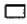is defined as follows: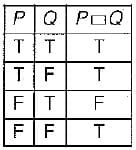Which one of the following is equivalent to P v Q?

Solution:

The given table can be converted into boolean function by adding mrnterms corresponding to true rows.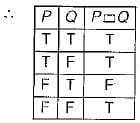Since there is only one false in the above truth table, we can represent the function PQ more efficiently, in conjunctive normal form.
Translates PQ = P + Q' (the max -term corresponding to the third row, where the function is false).
Now, we can easily translate the choices into boolean algebra as follows: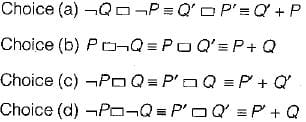As we can clearly see only choice (b)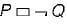is equivalent to P+ Q.

QUESTION: 8

Consider the following well-formed formulae: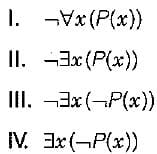Which of the above are equivalent?

Solution: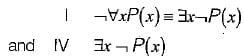Clearly, choices I and IV are equivalent.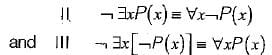Clearly II and III are not equivalent to each other or to I and IV.

QUESTION: 9

Which one of the following is NOT logically e quivalent to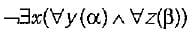Solution:
QUESTION: 10

The CORRECT formula for the sentence, "not all rainy days are cold” is

Solution:

In other words it says ``Some rainy days are not cold"

Given statement is
¬∀d[R(d)→C(d)]

≡¬∀d[¬R(d)∨C(d)
≡∃d[R(d)∧¬C(d)]Use Code STAYHOME200 and get INR 200 additional OFF Use Coupon Code